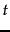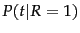Next: Review of basic probability Up: irbook Previous: Exercises   Contents   Index

Probabilistic information retrieval

During the discussion of relevance feedback in Section 9.1.2 , we observed that if we have some known relevant and nonrelevant documents, then we can straightforwardly start to estimate the probability of a termappearing in a relevant document, and that this could be the basis of a classifier that decides whether documents are relevant or not. In this chapter, we more systematically introduce this probabilistic approach to IR, which provides a different formal basis for a retrieval model and results in different techniques for setting term weights.

Users start with information needs, which they translate into query representations. Similarly, there are documents, which are converted into document representations (the latter differing at least by how text is tokenized, but perhaps containing fundamentally less information, as when a non-positional index is used). Based on these two representations, a system tries to determine how well documents satisfy information needs. In the Boolean or vector space models of IR, matching is done in a formally defined but semantically imprecise calculus of index terms. Given only a query, an IR system has an uncertain understanding of the information need. Given the query and document representations, a system has an uncertain guess of whether a document has content relevant to the information need. Probability theory provides a principled foundation for such reasoning under uncertainty. This chapter provides one answer as to how to exploit this foundation to estimate how likely it is that a document is relevant to an information need.

There is more than one possible retrieval model which has a probabilistic basis. Here, we will introduce probability theory and the Probability Ranking Principle (Sections 11.1 -11.2 ), and then concentrate on the Binary Independence Model (Section 11.3 ), which is the original and still most influential probabilistic retrieval model. Finally, we will introduce related but extended methods which use term counts, including the empirically successful Okapi BM25 weighting scheme, and Bayesian Network models for IR (Section 11.4 ). In Chapter 12 , we then present the alternative probabilistic language modeling approach to IR, which has been developed with considerable success in recent years.

SubsectionsNext: Review of basic probability Up: irbook Previous: Exercises   Contents   Index